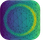#adaptive

Adaptive: parallel active learning of mathematical functions.

import os

import matplotlib.tri as mtri
import numpy as np
from matplotlib import animation
from matplotlib import pyplot as plt
from matplotlib.animation import FFMpegWriter
from PIL import Image, ImageDraw
from tqdm.auto import tqdm

# Make new images
draw = ImageDraw.Draw(circle)
alpha = Image.new("L", size, 0)

# Crop circles
w, h = size

# To array
cut = np.array(alpha)
cut = cut.reshape((*cut.shape, 1)).repeat(4, axis=2)

# Set the corners to (252, 252, 252, 255) to match the RTD background #FCFCFC
cut[:, :, -1] *= 255
cut[:, :, :-1] *= 252
return cut

def learner_till(till, learner, data):
new_learner.data = {k: v for k, v in data[:till]}
for x, y in learner._bounds_points:
# always include the bounds
new_learner.tell((x, y), learner.data[x, y])
return new_learner

def plot_tri(learner, ax):
tri = learner.ip().tri
triang = mtri.Triangulation(*tri.points.T, triangles=tri.vertices)
return ax.triplot(triang, c="k", lw=0.8, alpha=0.8)

def get_new_artists(npoints, learner, data, rounded_corners, ax):
new_learner = learner_till(npoints, learner, data)
line1, line2 = plot_tri(new_learner, ax)
data = np.rot90(new_learner.interpolated_on_grid()[-1])
im = ax.imshow(data, extent=(-0.5, 0.5, -0.5, 0.5), cmap="viridis")
im2 = ax.imshow(rounded_corners, extent=(-0.5, 0.5, -0.5, 0.5), zorder=10)
return im, line1, line2, im2

def create_and_run_learner():
def ring(xy):
import numpy as np

x, y = xy
a = 0.2
return x + np.exp(-((x**2 + y**2 - 0.75**2) ** 2) / a**4)

learner = adaptive.Learner2D(ring, bounds=[(-1, 1), (-1, 1)])
adaptive.runner.simple(learner, goal=lambda l: l.loss() < 0.005)
return learner

def main(fname="source/_static/logo_docs.mp4"):
learner = create_and_run_learner()

data = list(learner.data.items())

fig, ax = plt.subplots(figsize=(5, 5))
fig.subplots_adjust(left=0, bottom=0, right=1, top=1, wspace=None, hspace=None)
ax.set_xticks([])
ax.set_yticks([])
ax.spines["top"].set_visible(False)
ax.spines["right"].set_visible(False)
ax.spines["bottom"].set_visible(False)
ax.spines["left"].set_visible(False)

nseconds = 15
npoints = (len(data) * np.linspace(0, 1, 24 * nseconds) ** 2).astype(int)
artists = [
get_new_artists(n, learner, data, rounded_corners, ax) for n in tqdm(npoints)
]

ani = animation.ArtistAnimation(fig, artists, blit=True)
ani.save(fname, writer=FFMpegWriter(fps=24))

if __name__ == "__main__":
fname = "_static/logo_docs.mp4"
if not os.path.exists(fname):
main(fname)


adaptive is an open-source Python library designed to make adaptive parallel function evaluation simple. With adaptive you just supply a function with its bounds, and it will be evaluated at the “best” points in parameter space, rather than unnecessarily computing all points on a dense grid. With just a few lines of code you can evaluate functions on a computing cluster, live-plot the data as it returns, and fine-tune the adaptive sampling algorithm.

adaptive shines on computations where each evaluation of the function takes at least ≈100ms due to the overhead of picking potentially interesting points.

Run the adaptive example notebook live on Binder to see examples of how to use adaptive or visit the tutorial on Read the Docs.